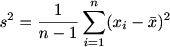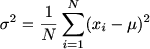Variance is used to measure the variability of the values in the dataset., Standard Deviation shows how spread out the numbers are, denoted by the symbol Sigma (σ).

A high variance describes the dataset which is more sprayed. On the other side, a low variance indicates that the dataset is highly clustered around the mean and spreads less.

Variance is defined as "The average squared differences from the mean." Using Variance, you can compute the standard Deviation.

Check out: Standard Deviation Calculator

### How to Use the Variance Calculator?

Step 1: Enter the numbers with a separate comma in the input box.

Step 2: Press the button to get the result.

Step 3: Check the result of your number and use it.

The best option to find the variance is to use our variance calculator.

### What is Meant by the Variance?

Mathematicians calculate Variance as the squared difference between the mean and the Variance calculated from the data set. To calculate Variance, follow the steps given below:

Step 1: Calculate the mean for the given set of data.

Step 2: Add the mean to each number and then square the result.

Step 3: Add together the squared differences to get the average.

For example,

5, 6, 7 are the set of data

To find variance,

Step 1: mean = (5 + 6 + 7)/3 = 18/3 = 6

Step 2: (5 – 6)2 + (6 – 6)2 + (7 – 6)2 = (-1)2 + 0 + (1)2

Step 3: (1 + 0 + 1)/3 = ⅔ = 0.667

### How to Find the Sample Variance and Population Variance?

Variance formulas

Varying values in a data set tell you how much they differ from the mean, a measure of dispersion. In other words, it's the average of the squares of the deviations from the norm. Squaring the deviations ensures that positive and negative deviations are not cancelled out.

The given sample variance calculator uses the following formula to calculate sample variance:

Formula 1: variance of a sampleHere, n is the sample size and x-bar is the sample mean

The given population variance calculator uses the following formula to calculate variance:

Formula 2: variance of the entire populationHere, N is the population size and μ is the population mean.

No need to go for long calculations when you’ve our population variance calculator in your hands.

You can easily use this variation calculator to find the variance.

History